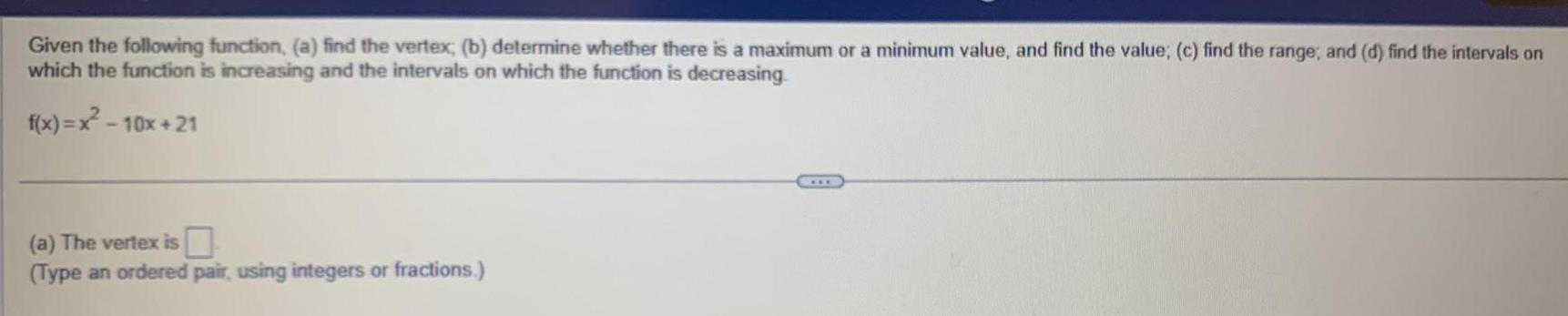Question:

# Given the following function a find the vertex b determine

Last updated: 9/14/2023Given the following function a find the vertex b determine whether there is a maximum or a minimum value and find the value c find the range and d find the intervals on which the function is increasing and the intervals on which the function is decreasing f x x 10x 21 a The vertex is Type an ordered pair using integers or fractions